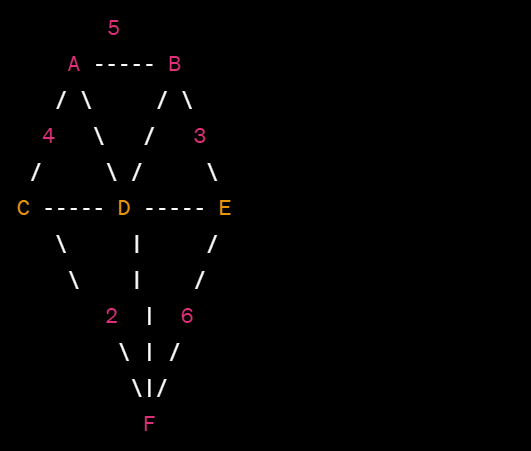Open In App

# GATE | GATE-CS-2001 | Question 39

Consider the following graph:Graph

Which edges would be included in the minimum spanning tree using Prim’s algorithm starting from vertex A?

Options: a)  b)  c)  d)

(A)

AB, BD, DE, EF, FC

(B)

AC, CD, DE, EB, BF

(C)

AB, BD, DE, EC, CF

(D)

AC, CD, DE, EB, FE

Explanation:

Let’s go through the steps of Prim’s algorithm starting from vertex A:

Start from vertex A and initialize the MST with just vertex A.
Find the minimum-weight edge that connects a vertex in the MST to a vertex outside the MST. The possible edges are AB (weight 5) and AC (weight 4).
Choose the minimum-weight edge, which is AC (weight 4), and add it to the MST.
Now, the MST includes vertices A and C. Look for the minimum-weight edge that connects a vertex in the MST to a vertex outside the MST. The possible edges are BC (weight 2), BD (weight 3), and CD (weight 6).
Choose the minimum-weight edge, which is BC (weight 2), and add it to the MST.
Now, the MST includes vertices A, B, and C. Look for the minimum-weight edge that connects a vertex in the MST to a vertex outside the MST. The possible edges are BD (weight 3), CD (weight 6), and DE (weight 3).
Choose the minimum-weight edge, which is BD (weight 3), and add it to the MST.
Now, the MST includes vertices A, B, C, and D. Look for the minimum-weight edge that connects a vertex in the MST to a vertex outside the MST. The possible edges are CD (weight 6), DE (weight 3), and EF (weight 5).
Choose the minimum-weight edge, which is DE (weight 3), and add it to the MST.
Now, the MST includes vertices A, B, C, D, and E. Look for the minimum-weight edge that connects a vertex in the MST to a vertex outside the MST. The possible edges are CD (weight 6), EF (weight 5), EC (weight 3), and FC (weight 2).
Choose the minimum-weight edge, which is FC (weight 2), and add it to the MST.
After going through all the steps, the minimum spanning tree (MST) using Prim’s algorithm starting from vertex A includes the following edges: AB, BC, BD, DE, and FC.

Therefore, the correct answer is option c) AB, BD, DE, EC, CF.

Quiz of this Question
Please comment below if you find anything wrong in the above post